login  home  contents  what's new  discussion  bug reports help  links  subscribe  changes  refresh  edit

 Submitted by : (unknown) at: 2007-11-17T22:04:33-08:00 (15 years ago) Name : Axiom Version : default friCAS-20090114 Axiom-20050901 OpenAxiom-20091012 OpenAxiom-20110220 OpenAxiom-Release-141 Category : Axiom Aldor Interface Axiom Compiler Axiom Library Axiom Interpreter Axiom Documentation Axiom User Interface building Axiom from source lisp system MathAction Doyen CD Reduce Axiom on Windows Axiom on Linux Severity : critical serious normal minor wishlist Status : open closed rejected not reproducible fix proposed fixed somewhere duplicate need more info Optional subject :   Optional comment :

The append function in axiom has the lisp background. axiom and lisp don't duplicate the last list.

fricas
(1) -> L1 := [1,2,3] ;
Type: List(PositiveInteger?)
fricas
L2 := [10,11,12] ;
Type: List(PositiveInteger?)
fricas
L := append (L1, L2) ;
Type: List(PositiveInteger?)
fricas
L2.2 := 100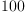(1)
Type: PositiveInteger?
fricas
L.5 -- is 100, not 11(2)
Type: PositiveInteger?

There is also a concat function that I understand in a mathematical way. today concat works as append.

fricas
L1 := [1,2,3];
Type: List(PositiveInteger?)
fricas
L2 := [10,11,12] ;
Type: List(PositiveInteger?)
fricas
Lc := concat (L1, L2)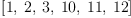(3)
Type: List(PositiveInteger?)
fricas
L1.2 :=20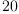(4)
Type: PositiveInteger?
fricas
Lc.2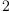(5)
Type: PositiveInteger?
fricas
L2.2 :=30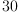(6)
Type: PositiveInteger?
fricas
Lc.5(7)
Type: PositiveInteger?

Lc doesn't change if I change L1, and Lc change if I modify L2. Is it possible to change concat in order to copy L2, as concat (L1, L2) == append (L1, copy (L2))

The concat function becomes just a little slower. And then list are here a real hi-level n-uple oblject, not a lisp object.

F.Maltey

Category: Axiom Compiler => Axiom Library

Status: open => closed

This is a common idiom and List is not "mathematical" but a data structure.

 Subject:   Be Bold !! ( 14 subscribers )